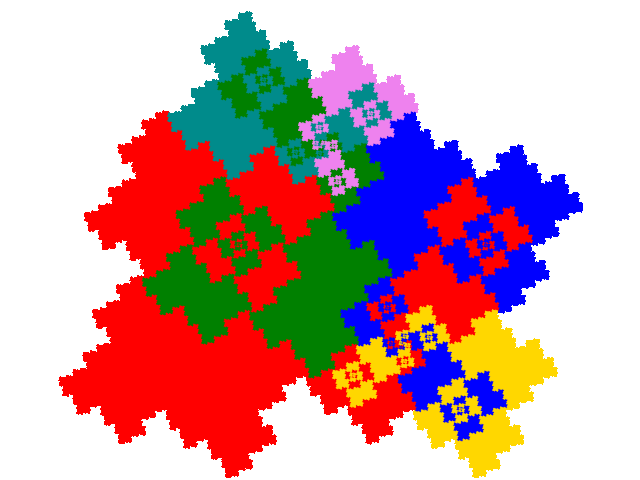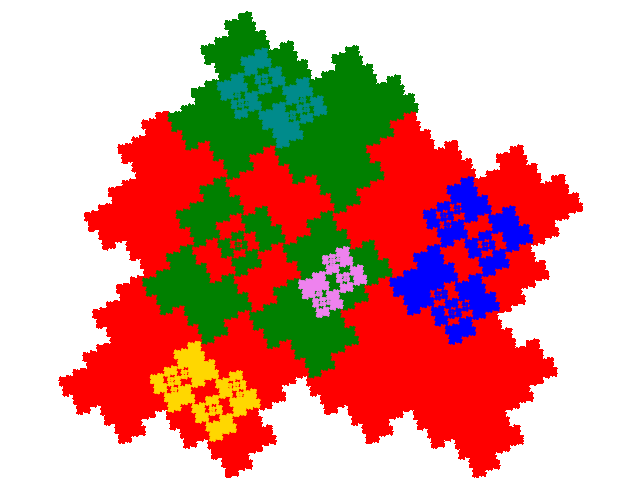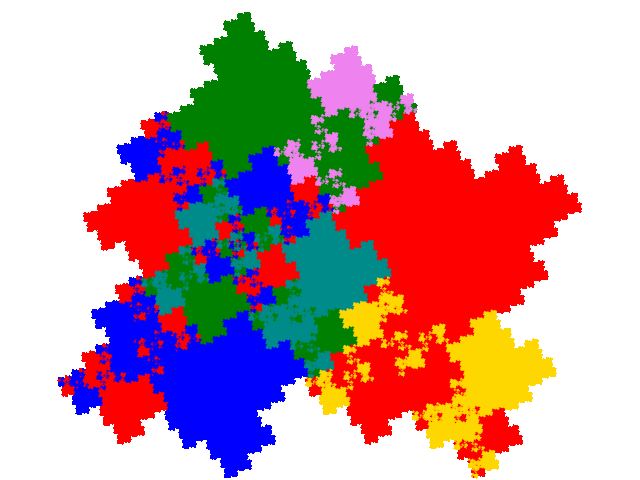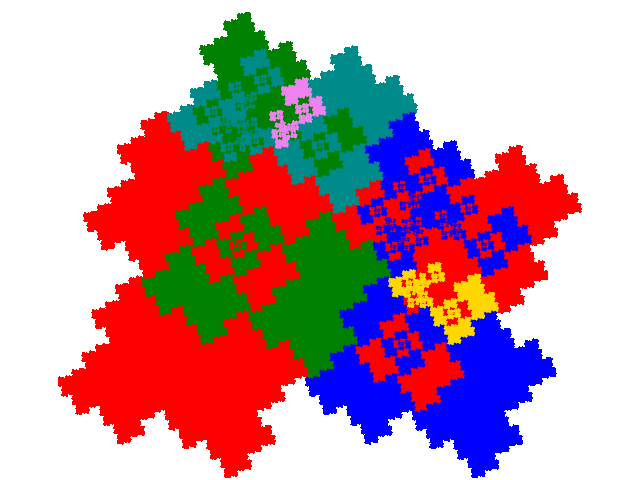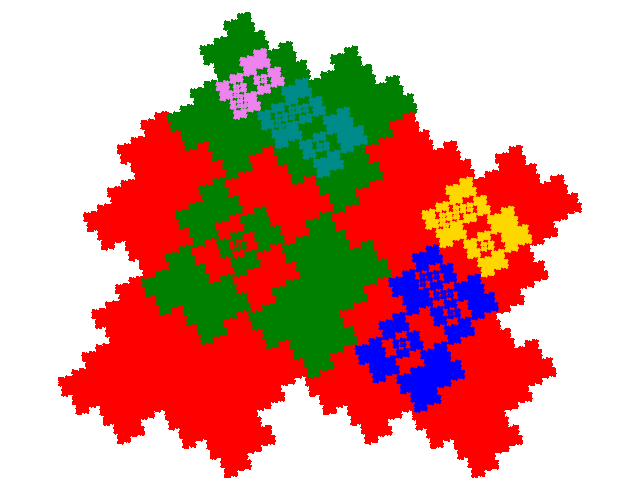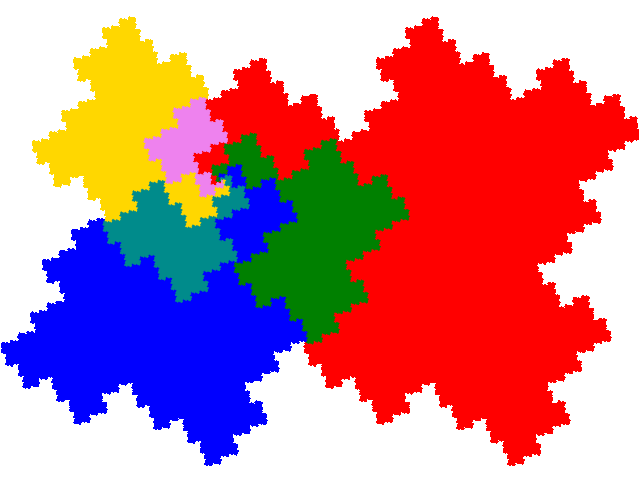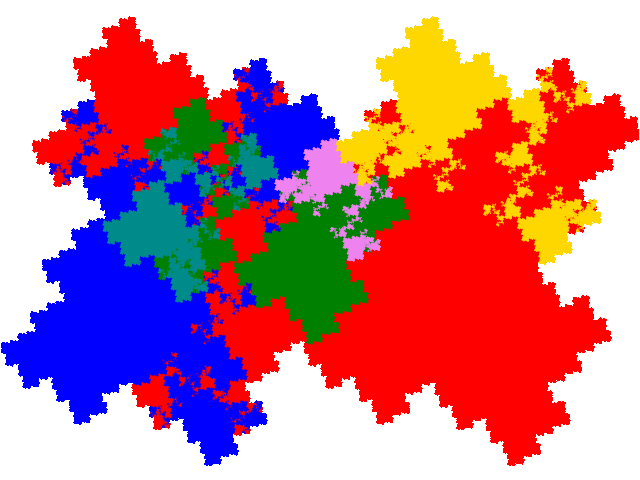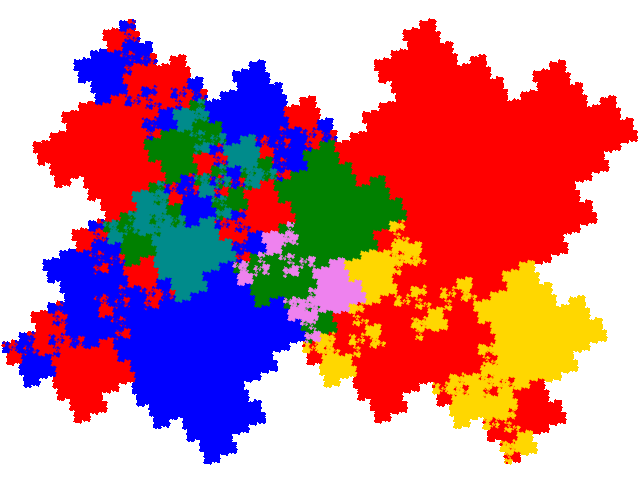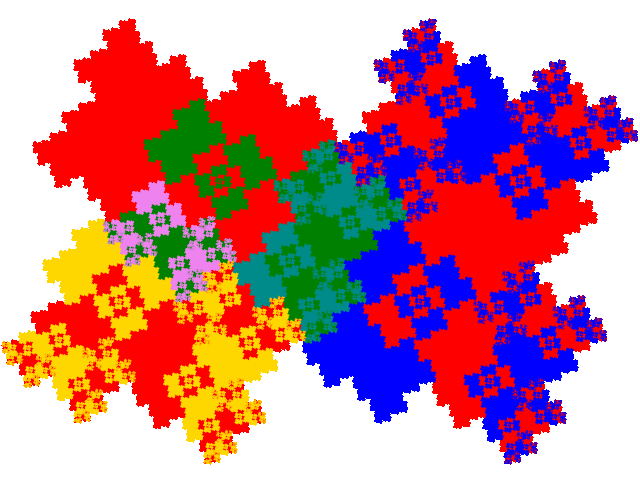# Some tiles associated with the 6th unit cubic Pisot number

## Allowindowed Tiles

Each normal order 3 tile has 9 potential legitimate order 7 partial post(allo)composition derivatives. However, as there are two distinct dissections of the windowed tile, this is increased to a potential 18 order 7 allodemisymmetric tiles. However 6 of the attractors are disconnected, and 2 are alternative dissections of the same attractor, reducing the number to 11.

As the windowed tile is a complex teragon, all the derived tiles are complex teragons.

For the first dissection tile there are 6 derived tiles. Four have the dissection equation c + c2 + 3c3 + c4 + c5 = 1 and tiling signature 001122, 011223 or 012234. (The windowed tile has alternative tilings with signatures 00, 01 and 02.)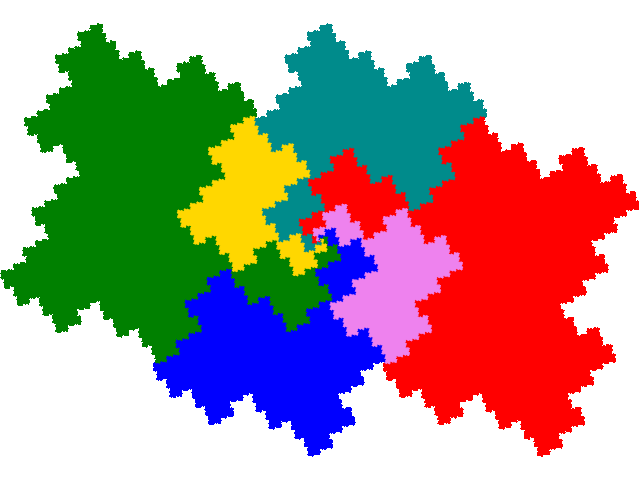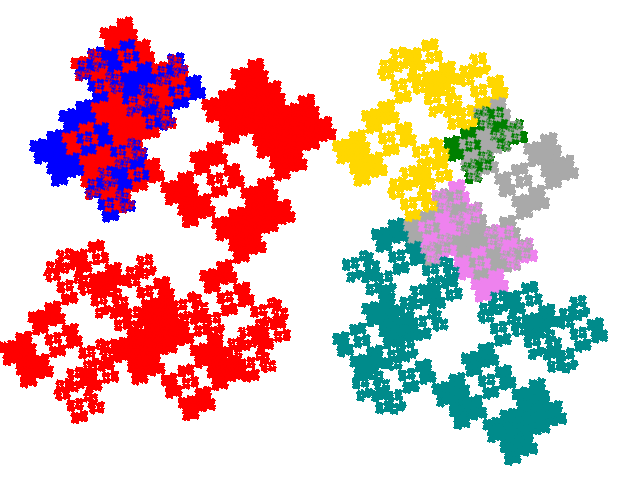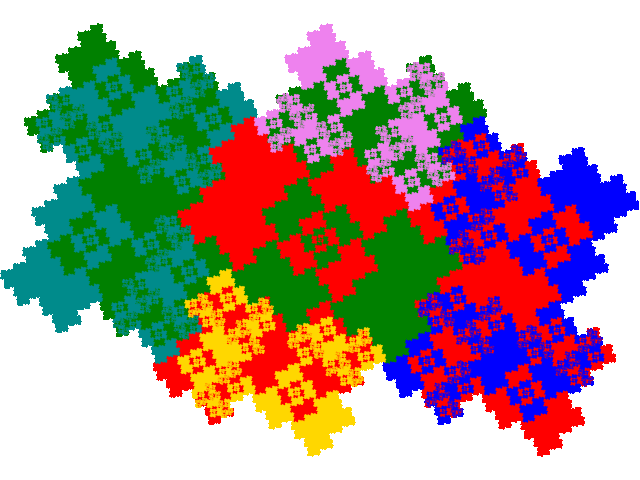Two have dissection equation 2c + c4 + 2c5 + c6 + c7, and tiling signature 003344, 013445 or 023456.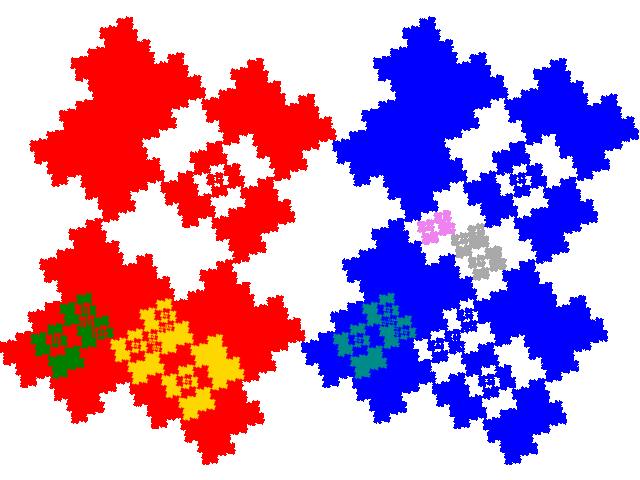The second dissection also has 6 derived tiles. The first is the alternative dissection of the first of the tiles above. Of the remainder two have dissection equation c + c2 + 3c3 + c4 + c5 = 1 and tiling signature 001122, 011223 or 012234.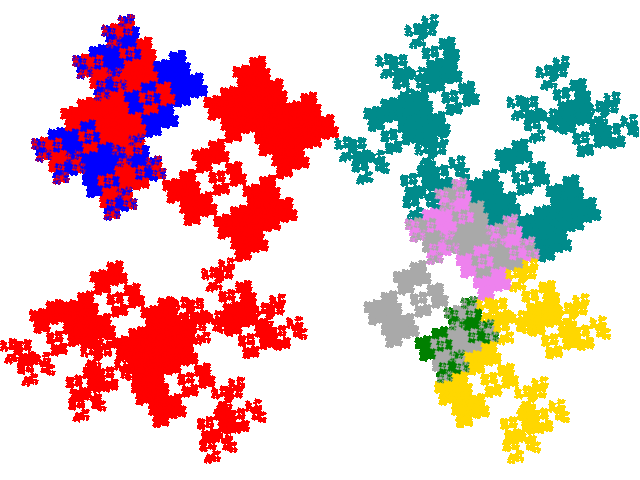One has dissection equation c + 2c2 + c3 + 2c5 + c7, and tiling signature 001144, 011245 or 012345.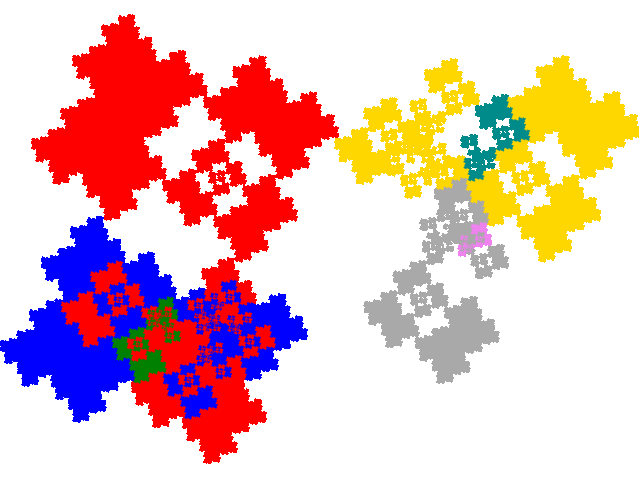and two have dissection equation 2c + c4 + 2c5 + c6 + c7, and tiling signature 003344, 013445 or 023456.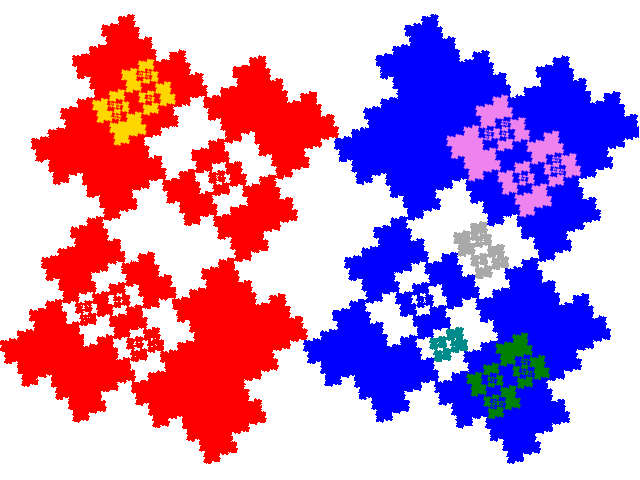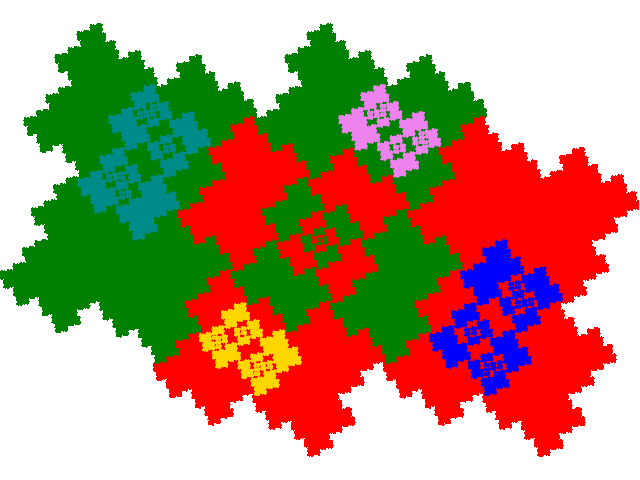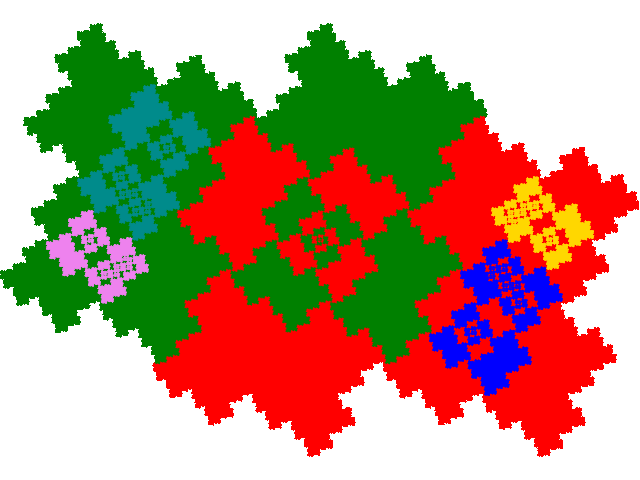The alternative unit cells are